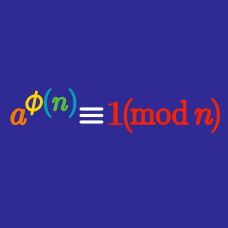Number Theory

# Euler's Theorem: Level 3 Challenges

How many positive integers $n>1$ evenly divide $a^{13}-a$ for all positive integers $a?$

Find the sum of all prime numbers $p$ that divides $\underset{\text{the digit 1 is repeated }p \text{ times}}{\underbrace{111111111111111\cdots 1}}$.

$\Huge {\color{#3D99F6}9}^{{\color{#20A900}8}^{{\color{#D61F06}7}^{{\color{#624F41}6} ^{\color{magenta}5}}}}$

What are the last two digits when this integer fully expanded out?

Let $P$ be product of all positive integers less than 720 which are relatively prime to 720. What is the remainder when $P^2$ is divided by 720?

Find the tens digit of $\Large 2014^{2014^{2014}}.$

×